x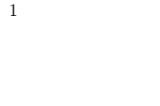Bell numberEncyclopedia
In combinatorics
Combinatorics
Combinatorics is a branch of mathematics concerning the study of finite or countable discrete structures. Aspects of combinatorics include counting the structures of a given kind and size , deciding when certain criteria can be met, and constructing and analyzing objects meeting the criteria ,...

, the nth Bell number, named after Eric Temple Bell
Eric Temple Bell
Eric Temple Bell , was a mathematician and science fiction author born in Scotland who lived in the U.S. for most of his life...

, is the number of partitions
Partition of a set
In mathematics, a partition of a set X is a division of X into non-overlapping and non-empty "parts" or "blocks" or "cells" that cover all of X...

of a set with n members, or equivalently, the number of equivalence relation
Equivalence relation
In mathematics, an equivalence relation is a relation that, loosely speaking, partitions a set so that every element of the set is a member of one and only one cell of the partition. Two elements of the set are considered equivalent if and only if they are elements of the same cell...

s on it. Starting with B0 = B1 = 1, the first few Bell numbers are:
1, 1, 2, 5, 15
15 (number)
15 is the natural number following 14 and preceding 16. In English, it is the smallest natural number with seven letters in its spelled name....

, 52
52 (number)
52 is the natural number following 51 and preceding 53.-In mathematics:Fifty-two is the 6th Bell number and a decagonal number...

, 203
203 (number)
203 is the natural number following 202 and preceding 204. It may be written as "two hundred three" or "two hundred and three".-In mathematics:203 is the 6th Bell number, i.e. it is the number of partitions of a set of size 6...

, 877, 4140, 21147, 115975, … .

## Partitions of a set

In general, Bn is the number of partitions
Partition of a set
In mathematics, a partition of a set X is a division of X into non-overlapping and non-empty "parts" or "blocks" or "cells" that cover all of X...

of a set of size n. A partition of a set S is defined as a set of nonempty, pairwise disjoint subsets of S whose union is S. For example, B3 = 5 because the 3-element set {abc} can be partitioned in 5 distinct ways:
{ {a}, {b}, {c} }
{ {a}, {b, c} }
{ {b}, {a, c} }
{ {c}, {a, b} }
{ {a, b, c} }.

B0 is 1 because there is exactly one partition of the empty set
Empty set
In mathematics, and more specifically set theory, the empty set is the unique set having no elements; its size or cardinality is zero. Some axiomatic set theories assure that the empty set exists by including an axiom of empty set; in other theories, its existence can be deduced...

. Every member of the empty set is a nonempty set (that is vacuously true
Vacuous truth
A vacuous truth is a truth that is devoid of content because it asserts something about all members of a class that is empty or because it says "If A then B" when in fact A is inherently false. For example, the statement "all cell phones in the room are turned off" may be true...

), and their union is the empty set. Therefore, the empty set is the only partition of itself.

Note that, as suggested by the set notation above, we consider neither the order of the partitions nor the order of elements within each partition. This means the following partitionings are all considered identical:
{ {b}, {a, c} }
{ {a, c}, {b} }
{ {b}, {c, a} }
{ {c, a}, {b} }.

## Another view of Bell numbers

Bell numbers can also be viewed as the number of distinct possible ways of putting n distinguishable balls into one or more indistinguishable boxes. For example, let us suppose n is 3. We have three balls, which we will label a, b, and c, and three boxes. If the boxes can not be distinguished from each other, there are five ways of putting the balls in the boxes:
• All three balls go in to one box. Since the boxes are anonymous, this is only considered one combination.
• a goes into one box; b and c go in to another box.
• b goes into one box; a and c go in to another box.
• c goes into one box; a and b go in to another box.
• Each ball goes in to its own box.

## Properties of Bell numbers

The Bell numbers satisfy this recursion formula: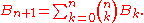They also satisfy "Dobinski's formula":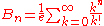= the nth moment
Moment (mathematics)
In mathematics, a moment is, loosely speaking, a quantitative measure of the shape of a set of points. The "second moment", for example, is widely used and measures the "width" of a set of points in one dimension or in higher dimensions measures the shape of a cloud of points as it could be fit by...

of a Poisson distribution
Poisson distribution
In probability theory and statistics, the Poisson distribution is a discrete probability distribution that expresses the probability of a given number of events occurring in a fixed interval of time and/or space if these events occur with a known average rate and independently of the time since...

with expected value
Expected value
In probability theory, the expected value of a random variable is the weighted average of all possible values that this random variable can take on...

1.

And they satisfy "Touchard's congruence": If p is any prime number
Prime number
A prime number is a natural number greater than 1 that has no positive divisors other than 1 and itself. A natural number greater than 1 that is not a prime number is called a composite number. For example 5 is prime, as only 1 and 5 divide it, whereas 6 is composite, since it has the divisors 2...

then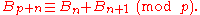or, generalizing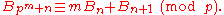Each Bell number is a sum of Stirling numbers of the second kind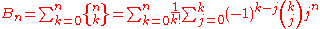The Stirling number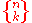is the number of ways to partition a set of cardinality n into exactly k nonempty subsets.

More generally, the Bell numbers satisfy the following recurrence: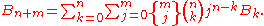The nth Bell number is also the sum of the coefficients in the polynomial that expresses the nth moment
Moment (mathematics)
In mathematics, a moment is, loosely speaking, a quantitative measure of the shape of a set of points. The "second moment", for example, is widely used and measures the "width" of a set of points in one dimension or in higher dimensions measures the shape of a cloud of points as it could be fit by...

of any probability distribution
Probability distribution
In probability theory, a probability mass, probability density, or probability distribution is a function that describes the probability of a random variable taking certain values....

as a function of the first n cumulant
Cumulant
In probability theory and statistics, the cumulants κn of a probability distribution are a set of quantities that provide an alternative to the moments of the distribution. The moments determine the cumulants in the sense that any two probability distributions whose moments are identical will have...

s; this way of enumerating partitions is not as coarse as that given by the Stirling numbers.

The exponential generating function
Generating function
In mathematics, a generating function is a formal power series in one indeterminate, whose coefficients encode information about a sequence of numbers an that is indexed by the natural numbers. Generating functions were first introduced by Abraham de Moivre in 1730, in order to solve the general...

of the Bell numbers is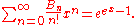## Asymptotic limit and bounds

Several asymptotic formulae for the Bell numbers are known. One such is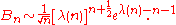Here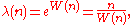where W is the Lambert W function.

(Lovász
László Lovász
László Lovász is a Hungarian mathematician, best known for his work in combinatorics, for which he was awarded the Wolf Prize and the Knuth Prize in 1999, and the Kyoto Prize in 2010....

, 1993)

In (Berend, D. and Tassa, T., 2010), the following bounds were established: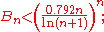moreover, if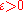then for all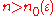,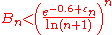where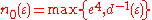and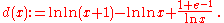## Triangle scheme for calculating Bell numbers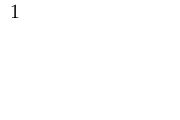The Bell numbers can easily be calculated by creating the so-called Bell triangle, also called Aitken's array or the Peirce triangle:
1. Start with the number one. Put this on a row by itself.
2. Start a new row with the rightmost element from the previous row as the leftmost number
3. Determine the numbers not on the left column by taking the sum of the number to the left and the number above the number to the left (the number diagonally up and left of the number we are calculating)
4. Repeat step three until there is a new row with one more number than the previous row
5. The number on the left hand side of a given row is the Bell number for that row.

For example, the first row is made by placing one by itself. The next (second) row is made by taking the rightmost number from the previous row (1), and placing it on a new row. We now have a structure like this:

```
1
1  x
```

The value x here is determined by adding the number to the left of x (one) and the number above the number to the left of x (also one).

```
1
1  2
y
```

The value y is determined by copying over the number from the right of the previous row. Since the number on the right hand side of the previous row has a value of 2, y is given a value of two.

```
1
1  2
2  3  x
```

Again, since x is not the leftmost element of a given row, its value is determined by taking the sum of the number to x's left (three) and the number above the number to x's left (two). The sum is five.

Here is the first five rows of this triangle:

```
1
1  2
2  3  5
5  7 10 15
15 20 27 37 52
```

The fifth row is calculated thus:
• Take 15 from the previous row
• 15 + 5 = 20
• 20 + 7 = 27
• 27 + 10 = 37
• 37 + 15 = 52

### Computer program

The following is example code in the Ruby programming language that prints out all the Bell numbers from the 1st to the 300th inclusive (the limits can be adjusted)

1. !/usr/bin/env ruby

def print_bell_numbers(start, finish)
# Initialize the Bell triangle as a two-dimensional array
triangle = Array[Array]

# Make sure "start" is less than "finish", and both numbers are at least 1
(finish, start = start, finish) if finish < start
start = 1 if start < 1
finish = 1 if finish < 1

1.upto(finish-1) do |row_num|

# Set the first element of the current row to be the last element
# of the previous row
current_row = [triangle[row_num-1][row_num-1]]

# Calculate the rest of the elements in this row, then add the row
# to the Bell triangle
1.upto(row_num) do |col_num|
sum = triangle[row_num-1][col_num-1] + current_row[col_num-1]
current_row.push(sum)
end

triangle[row_num] = current_row

end

# Print out the Bell numbers
start.upto(finish) do |num|
puts triangle[num-1]
end
end

print_bell_numbers(1, 300)

And here an equivalent version written in Python
Python (programming language)
Python is a general-purpose, high-level programming language whose design philosophy emphasizes code readability. Python claims to "[combine] remarkable power with very clear syntax", and its standard library is large and comprehensive...

def bell_numbers(start, stop):
## Swap start and stop if start > stop
if stop < start: start, stop = stop, start
if start < 1: start = 1
if stop < 1: stop = 1

t = 1
1
Year 1 was a common year starting on Saturday or Sunday of the Julian calendar and a common year starting on Saturday of the Proleptic Julian calendar...

## Initialize the triangle as a two-dimensional array
c = 1 ## Bell numbers count
while c <= stop:
if c >= start:
yield t[-1] ## Yield the Bell number of the previous row
row = [t[-1][-1]] ## Initialize a new row
for b in t[-1]:
row.append(row[-1] + b) ## Populate the new row
c += 1 ## We have found another Bell number
t.append(row) ## Append the row to the triangle

for b in bell_numbers(1, 300):
print b

The number in the nth row and kth column is the number of partitions of {1, ..., n} such that n is not together in one class with any of the elements k, k + 1, ..., n − 1. For example, there are 7 partitions of {1, ..., 4} such that 4 is not together in one class with either of the elements 2, 3, and there are 10 partitions of {1, ..., 4} such that 4 is not together in one class with element 3. The difference is due to 3 partitions of {1, ..., 4} such that 4 is together in one class with element 2, but not with element 3. This corresponds to the fact that there are 3 partitions of {1, ..., 3} such that 3 is not together in one class with element 2: for counting partitions two elements which are always in one class can be treated as just one element. The 3 appears in the previous row of the table.

## Prime Bell numbers

The first few Bell numbers that are primes are:
2, 5, 877, 27644437, 35742549198872617291353508656626642567, 359334085968622831041960188598043661065388726959079837

corresponding to the indices 2, 3, 7, 13, 42 and 55 .

The next prime is B2841, which is approximately 9.30740105 × 106538. http://primes.utm.edu/primes/page.php?id=68825 , it is the largest known prime Bell number. Phil Carmody showed it was a probable prime
Probable prime
In number theory, a probable prime is an integer that satisfies a specific condition also satisfied by all prime numbers. Different types of probable primes have different specific conditions...

in 2002. After 17 months of computation with Marcel Martin's ECPP
Elliptic curve primality proving
Elliptic Curve Primality Proving is a method based on elliptic curves to prove the primality of a number . It is a general-purpose algorithm, meaning it does not depend on the number being of a special form...

program Primo, Ignacio Larrosa Cañestro proved it to be prime in 2004. He ruled out any other possible primes below B6000, later extended to B30447 by Eric Weisstein.